## Lift

Given a Mapfrom a Space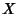to a Space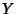and another Mapfrom a Space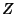to a Space, a lift is a Mapfromtosuch that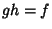. In other words, a lift ofis a Mapsuch that the diagram (shown below) commutes.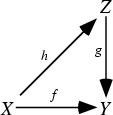Ifis the identity fromto, a Manifold, and ifis the bundle projection from the Tangent Bundle to, the lifts are precisely Vector Fields. Ifis a bundle projection from any Fiber Bundle to, then lifts are precisely sections. Ifis the identity fromto, a Manifold, anda projection from the orientation double cover of, then lifts exist Iffis an orientable Manifold.

Ifis a Map from a Circle to, an-Manifold, andthe bundle projection from the Fiber Bundle of alternating k-Form on, then lifts always exist Iffis orientable. Ifis a Map from a region in the Complex Plane to the Complex Plane (complex analytic), and ifis the exponential Map, lifts ofare precisely Logarithms of.